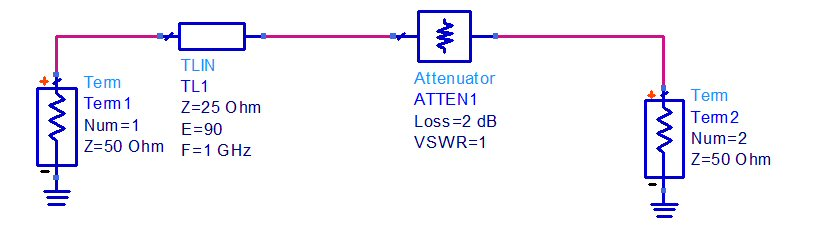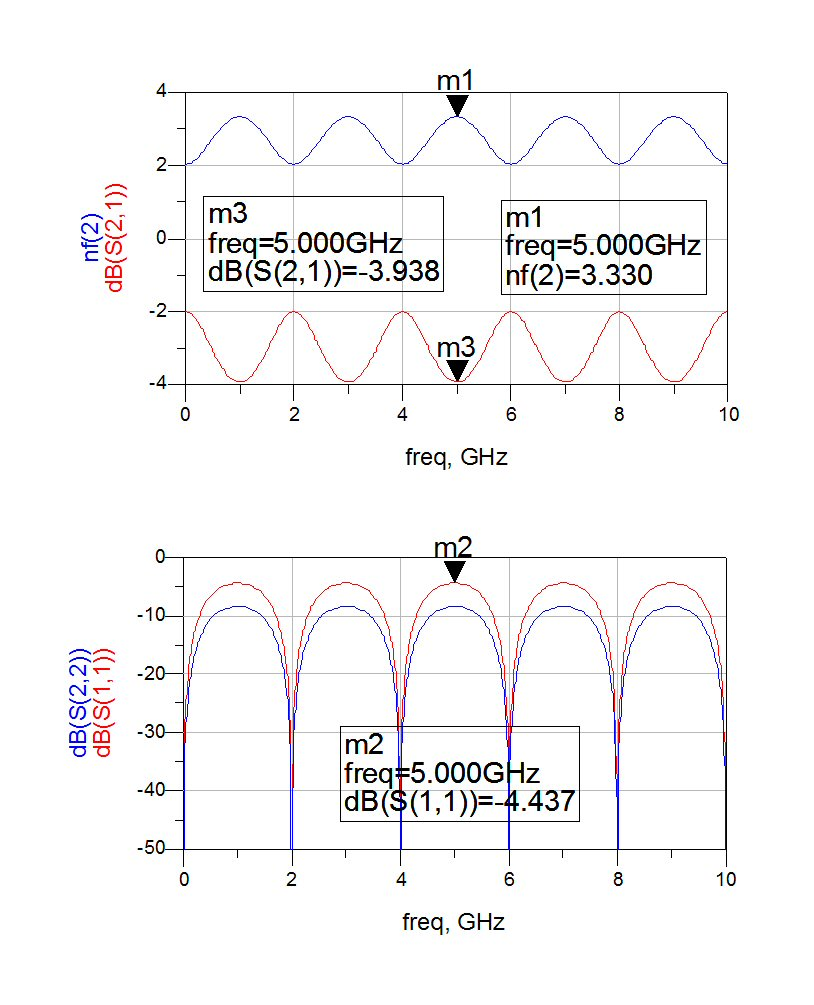# Mixer Noise Figure

New for February 2012! This page is kind of a mess, because the content is controversial (in that not eveyone agrees) so take it all with a grain of salt for now. We appreciate anyone that want to kick in some knowledge on the topic, especially if they include some references...

On this page we will post some information on the noise figure of a mixer. We posted a a rule of thumb, many years ago, that states:

Mixer noise figure is "roughly equal to the magnitude of its conversion loss, or just a little bit less. For example, a mixer with -6 dB conversion gain might have 5.5 dB noise figure".

In support of our Rule of Thumb:

What if the conversion loss was partially due to bad reflection coefficient? If the mixer had 3:1 mismatch at the RF port, mismatch loss would be 1.25 dB. That 1.25 dB would not increase the signal to noise ratio, the way I understand it, but I could be wrong. I have had other people disagree with this, maybe you are right and I am wrong…. and I have seen LNAs with great NF but really bad S11, which supports my understanding of the problem. What about an antenna element with 3:1 mismatch? It drops the gain of the receiver but doesn't affact the noise figure.

I recall measuring mixers and seeing NF less than conversion loss a long time ago, mixers with bad return loss.

Here's a 2 dB attenuator that suffers a mismatch on the input, due to a 25 ohm 1 GHz quarter-wave transformer that mismatches the circuit at 1, 3, 5, 7... GHz:And below the noise figure and S-parameters. At the worst frequencies a 4:1 VSWR is created (you are looking into 12.5 ohms in 50 ohm system). Calculating the mismatch loss (see our VSWR calulator), you get 1.938 dB. The transmission coefficient is -3.938 (see marker). But what about the noise figure? It simulates at 3.33 dB, which is less than the insertion loss of the network... hmmm...From Roy:

There are a lot of subtle issues to consider when you're dealing with noise that is not only an input to a circuit but also generated in all of the lossy components. Your example of the mismatch loss might not degrade (increase) the signal to noise ratio as you say, but I don't believe it would improve it either. Typically the way it is viewed (from my perspective) is that you have a noise figure associated with the signal loss (conversion loss) through the mixer. Let's assume for this argument that we are talking about a double sideband noise figure, so that we aren't penalized by the noise folding on itself. If you had a lossless mixer, then the noise figure should be equal to the conversion loss, but there is going to be some degradation of the noise figure due to internally generated excess noise (above KTB) in the mixer. That's why my opinion would be that the noise figure of a mixer is always higher than its conversion loss.

From "The Professor"

I'll look into this again. I seem to recall that somehow it is possible for mixer noise figure to be somewhat less than its conversion loss. And Roy's right, that does imply that its output noise power density is less than kTo, or -174 dBm / Hz. In fact, back in 2002 or 2003, we measured a mixer that had a lower noise figure (measured) than its conversion loss, and we looked into it then. I think one of us had found an app note or a paper about that at the time.

I think one way for the output noise to be less than kTo in the IF band is, the input noise power density (which is assumed to be kTo in the definition of noise figure) doesn't all get converted to the IF band in the ideal mixer. At least half gets converted to the other (undesired) sideband, e.g., the USB if the mixer is being used as a downconverter. And some gets converted to harmonics, so ideally you get (I think) about a 3.9 dB conversion loss from an ideal diode mixer. In that mixer, since the same amount of the input noise power is converted to the undesired sideband and harmonics as the input signal, you'd have a 0 dB noise figure - output SNR equal to input SNR. In the real world the diodes themselves introduce some noise, so the noise figure could be equal to or even greater than the loss in a real mixer. But I think the possibility is still open for the noise figure to be lower than the loss, too.

There seems to be a common misconception in the industry that the noise figure of any passive, lossy part is always equal to its loss. The truth is, that's only true when all the loss comes from dissipation and the part's temperature is exactly 290 Kelvin. If some of the loss comes from mismatch, or the part is colder than 290 K, the noise figure is lower than the loss, and if the part's hotter than 290 K, the noise figure can be higher.

I think there's probably another misconception that kTo (-174 dBm / Hz) is some kind of "floor" for noise power density, like it can never be lower than that. But it's easy to think of a signal source with lower noise density. Imagine an antenna that's highly efficient (gain divided by directivity is almost one), or maybe it's cryogenically cooled. And this antenna is pointing at a "cool" region in the sky, away from the sun, the moon, or the galactic center. Then the antenna noise temperature will be only about 4 K, so its output noise density would be somewhere around -193 dBm / Hz.

From Don:

Here is something to consider. In an ideal diode, the shot noise, when heavily forward-biased, will be kTB/2. That’s right, with heavy forward bias an ideal diode makes less noise than kTB. When I was a youngster and first learned that, I thought “I’m gonna use that to build a solid-state refrigerator!”. But, that turns out to be impractical.

So, it is conceivable that a mixer’s noise figure could be less than its loss, to me anyway.

## Measuring mixer noise figure

Just when you think this all makes sense, go out in the lab and try to measure noise figure of a mixer... you'll soon see there are things that require explanation, other than "the measurement was suspicious". On the other hand, in most receivers, mixer NF is buried after the LNA and is not so important that you need 100% accurate data.

From "The Professor"

We had measured an NF that was lower than the loss in a mixer. I'm pretty sure it was an L-band, single-diode mixer. Our measurements were really suspect because this mixer's conversion loss was higher than its LO to IF isolation, and we were using a broadband noise source, like an HP346B. That meant that noise power at the IF, going into the LO input, was coming out the IF output. We had to use all kinds of filters around the mixer to get a decent measurement, and as I recall even with all the filters the noise figure was still a little lower than the loss.

Usually a lossy part loses the input power by dissipating it. And the dissipative part of the loss contributes to noise power, which is why "the noise figure is equal to the loss" is almost always true when the part is at 290K. I've seen mixers where the noise figure measures less than the conversion loss myself. In fact a guy we both know, when he was an intern, made some mixer measurements where the noise figure was a fair amount lower than the loss, and I had to do some thinking about how that could be the case. The best explanation I could come up with at the time was that a good part of a mixer's conversion loss comes from frequency spreading, i.e., some of the input signal leaks through to the output port without being changed in frequency, some leaks to the LO port the same way, some is converted to the wrong sideband, and some is converted to harmonics of the output frequency. And of course some of the loss comes from dissipation. Conversely, there is additional noise power generated by the mixer diodes, which could potentially increase the mixer's noise figure above the value of its loss. But I believe in a balanced mixer, a lot of that noise power cancels out.

Author : Unknown Editor